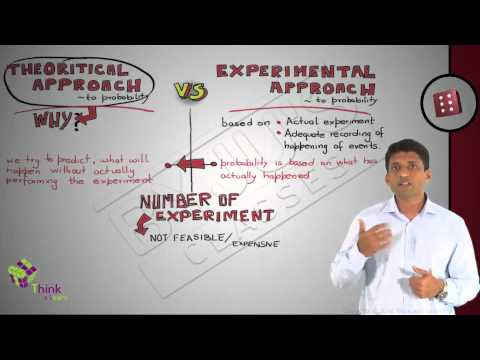# Experimental Probability

You and your 3 friends are playing a board game. It’s your turn to roll the die and to win the game you need a 5 on the dice. Now, is it possible that upon rolling the die you will get an exact 5? No, it is a matter of chance. We face multiple situations in real life where we have to take a chance or risk. Based on certain conditions, the chance of occurrence of a certain event can be easily predicted. In our day to day life, we are more familiar with the word ‘chance and probability’. In simple words, the chance of occurrence of a particular event is what we study in probability. In this article, we are going to discuss one of the types of probability called  “Experimental Probability” in detail.

## What is Probability?

Probability, a branch of Math that deals with the likelihood of the occurrences of the given event. The probability values for the given experiment is usually defined between the range of numbers. The values lie between the numbers 0 and 1. The probability value cannot be a negative value. The basic rules such as addition, multiplication and complement rules are associated with the probability.

## Experimental Probability Vs Theoretical Probability

There are two approaches to study probability:

• Experimental Probability
• Theoretical Probability

### What is Experimental Probability?

Experimental probability, also known as Empirical probability, is based on actual experiments and adequate recordings of the happening of events. To determine the occurrence of any event, a series of actual experiments are conducted. Experiments which do not have a fixed result are known as random experiments. The outcome of such experiments is uncertain. Random experiments are repeated multiple times to determine their likelihood. An experiment is repeated a fixed number of times and each repetition is known as a trial. Mathematically, the formula for the experimental probability is defined by;

Probability of an Event P(E) = Number of times an event occurs / Total number of trials.

### What is Theoretical Probability?

In probability, the theoretical probability is used to find the probability of an event. Theoretical probability does not require any experiments to conduct. Instead of that, we should know about the situation to find the probability of an event occurring. Mathematically, the theoretical probability is described as the number of favourable outcomes divided by the number of possible outcomes.

Probability of Event P(E) = No. of. Favourable outcomes/ No. of. Possible outcomes.

### Experimental Probability Example

Example: You asked your 3 friends Shakshi, Shreya and Ravi to toss a fair coin 15 times each in a row and the outcome of this experiment is given as below:

 Coin Tossed By: No. of. Heads No. of. Tails Shakshi 6 9 Shreya 7 8 Ravi 8 7

Calculate the probability of occurrence of heads and tails.

Solution: The experimental probability for the occurrence of heads and tails in this experiment can be calculated as:

Experimental Probability of Occurrence of heads = Number of times head occurs/Number of times coin is tossed.

Experimental Probability of Occurrence of tails = Number of times tails occurs/Number of times coin is tossed.

 Coin Tossed By: No. of. Heads No. of. Tails Experimental Probability for the occurrence of Head Experimental Probability for the occurrence of Tail Shakshi 6 9 6/15 = 0.4 9/15 = 0.6 Shreya 7 8 7/15 = 0.47 8/15 = 0.53 Ravi 8 7 8/15 = 0.53 7/15 = 0.47

We observe that if the number of tosses of the coin increases then the probability of occurrence of heads or tails also approaches to 0.5.